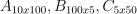/NumPy 1.17

# numpy.linalg.multi_dot

`numpy.linalg.multi_dot(arrays)` [source]

Compute the dot product of two or more arrays in a single function call, while automatically selecting the fastest evaluation order.

`multi_dot` chains `numpy.dot` and uses optimal parenthesization of the matrices  . Depending on the shapes of the matrices, this can speed up the multiplication a lot.

If the first argument is 1-D it is treated as a row vector. If the last argument is 1-D it is treated as a column vector. The other arguments must be 2-D.

Think of `multi_dot` as:

```def multi_dot(arrays): return functools.reduce(np.dot, arrays)
```
Parameters: `arrays : sequence of array_like` If the first argument is 1-D it is treated as row vector. If the last argument is 1-D it is treated as column vector. The other arguments must be 2-D. `output : ndarray` Returns the dot product of the supplied arrays.

See also

`dot`
dot multiplication with two arguments.

#### Notes

The cost for a matrix multiplication can be calculated with the following function:

```def cost(A, B):
return A.shape * A.shape * B.shape
```

Assume we have three matrices.

The costs for the two different parenthesizations are as follows:

```cost((AB)C) = 10*100*5 + 10*5*50   = 5000 + 2500   = 7500
cost(A(BC)) = 10*100*50 + 100*5*50 = 50000 + 25000 = 75000
```

#### References

  Cormen, “Introduction to Algorithms”, Chapter 15.2, p. 370-378

#### Examples

`multi_dot` allows you to write:

```>>> from numpy.linalg import multi_dot
>>> # Prepare some data
>>> A = np.random.random((10000, 100))
>>> B = np.random.random((100, 1000))
>>> C = np.random.random((1000, 5))
>>> D = np.random.random((5, 333))
>>> # the actual dot multiplication
>>> _ = multi_dot([A, B, C, D])
```

instead of:

```>>> _ = np.dot(np.dot(np.dot(A, B), C), D)
>>> # or
>>> _ = A.dot(B).dot(C).dot(D)
```

© 2005–2019 NumPy Developers
Licensed under the 3-clause BSD License.
https://docs.scipy.org/doc/numpy-1.17.0/reference/generated/numpy.linalg.multi_dot.html